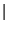IdentifiantMot de passe
Mot de passe oublié ?
11.4 Character array methods

# 11.4 Character array methods

CharArray object has these public methods:

 tolist( )
`tolist()` returns a nested list of strings corresponding to all the elements in the array.
 copy( )
`copy()` returns a deep copy of the character array.
 raw( )
`raw()` returns the corresponding `RawCharArray` view.
```     >>> c=str.array(["this","that","another"])
>>> c.raw()
RawCharArray(['this   ', 'that   ', 'another'])
```
 resized( n, fill=' ')
`resized(n)` returns a copy of the array, resized so that each element is of length `n` characters. Extra characters are filled with value `fill`. Caution: do not confuse this method with `resize()` which changes the number of elements rather than the size of each element.
```     >>> c = str.array(["this","that","another"])
>>> c.itemsize()
7
>>> d = c.resized(20)
>>> print d
['this', 'that', 'another']
>>> d.itemsize()
20
```
 concatenate( other)
`concatenate(other)` returns a new array which corresponds to the element by element concatenation of `other` to `self`. The addition operator is also overloaded to perform concatenation.
```     >>> print map(str, range(3)) + array(["this","that","another one"])
['0this', '1that', '2another one']
>>> print "prefix with trailing whitespace   " + array(["."])
['prefix with trailing whitespace   .']
```
 sort( )
`sort` modifies the `CharArray` inplace so that its elements are in sorted order. `sort` only works for 1D character arrays. Like the `sort()` for the Python list, `CharArray.sort()` returns nothing.
```     >>> a=str.array(["other","this","that","another"])
>>> a.sort()
>>> print a
['another', 'other', 'that', 'this']
```
 argsort( )
`argsort` returns a numarray corresponding to the permutation which will put the character array `self` into sorted order. `argsort` only works for 1D character arrays.
```     >>> a=str.array(["other","that","this","another"])
>>> a.argsort()
array([3, 0, 1, 2])
>>> print a[ a.argsort ]
['another', 'other', 'that', 'this']
```
 amap( f)
`amap` applies the function `f` to every element of `self` and returns the nested list of the results. The function `f` should operate on a single string and may return any Python value.
```     >>> c = str.array(['this','that','another'])
>>> print c.amap(lambda x: x[-2:])
['is', 'at', 'er']
```
 match( pattern, flags=0)
`match` uses Python regular expression matching over all elements of a character array and returns a tuple of numarrays corresponding to the indices of `self` where the pattern matches. `flags` are passed directly to the Python pattern matcher defined in the `re` module of the standard library.
```     >>> a=str.array([["wo","what"],["wen","erewh"]])
>>> print a.match("wh[aebd]")
(array(), array())
>>> print a[ a.match("wh[aebd]") ]
['what']
```
 search( pattern,flags=0)
`search` uses Python regular expression searching over all elements of a character array and returns a tuple of numarrays corresponding to the indices of `self` where the pattern was found. `flags` are passed directly to the Python pattern `search` method defined in the `re` module of the standard library. `flags` should be an or'ed combination (use theoperator) of the following `re` variables: `IGNORECASE`, `LOCALE`, `MULTILINE`, `DOTALL`, `VERBOSE`. See the `re` module documentation for more details.
 sub( pattern,replacement,flags=0,count=0)
`sub` performs Python regular expression pattern substitution to all elements of a character array. `flags` and `count` work as they do for `re.sub()`.
```     >>> a=str.array([["who","what"],["when","where"]])
>>> print a.sub("wh", "ph")
[['pho', 'phat'],
['phen', 'phere']])
```
 grep( pattern, flags=0)
`grep` is intended to be used interactively to search a `CharArray` for the array of strings which match the given `pattern`. `pattern` should be a Python regular expression (see the `re` module in the Python standard library, which can be as simple as a string constant as shown below.
```     >>> a=str.array([["who","what"],["when","where"]])
>>> print a.grep("whe")
['when', 'where']
```
 eval( )
`eval` executes the Python eval function on each element of a character array and returns the resulting numarray. `eval` is intended for use converting character arrays to the corresponding numeric arrays. An exception is raised if any string element fails to evaluate.
```     >>> print str.array([["1","2"],["3","4."]]).eval()
[[1., 2.],
[3., 4.]]
```
 maxLen( )
`maxLen` returns the minimum element length required to store the stripped elements of the array `self`.
```     >>> print str.array(["this","there"], itemsize=20).maxLen()
5
```
 truncated( )
`truncated` returns an array corresponding to `self` resized so that it uses a minimum amount of storage.
```     >>> a = str.array(["this  ","that"])
>>> print a.itemsize()
6
>>> print a.truncated().itemsize()
4
```
 count( s)
`count` counts the occurences of string `s` in array `self`.
```     >>> print array(["this","that","another","this"]).count("this")
2
```
 info( )
This will display key attributes of the character array.

Send comments to the NumArray community.